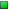Inconsistent Frame Rate
 Posted: 24 February 2011 12:37 PM [ Ignore ]
New MemberTotal Posts:  28
Joined  2011-01-15

My application is more sensitive to minimum camera frame rate than average frame rate.

Using the SDK, I have a loop with only the following:

cvGetImageRawData(pCapImage, &pCapBuffer;);

CLEyeCameraGetFrame(_cam, pCapBuffer);
tickFPS = ::GetTickCount();
timeFPS = tickFPS - oldTickFPS;
oldTickFPS = tickFPS;

fps = 1000.0f/((float)timeFPS);

if (fps

< tMin)
tMin = fps;

if(fps >

tMax)
tMax = fps;
tAvg = ((tAvg * cnt) + fps) / (cnt+1);
cnt += 1;

printf(“fps = %g\n “, fps);

With cam = new CLEyeCameraCapture(windowName, guid, CLEYE_COLOR_PROCESSED, CLEYE_VGA,30),  I see this (every 15th frame has 31.25 fps)

fps = 66.6667
fps = 62.5
fps = 66.6667
fps = 62.5
fps = 62.5
fps = 66.6667
fps = 62.5
fps = 62.5
fps = 66.6667
fps = 62.5
fps = 66.6667
fps = 62.5
fps = 62.5
fps = 66.6667
fps = 31.25
fps = 66.6667
fps = 62.5
fps = 66.6667
fps = 62.5
fps = 62.5
fps = 66.6667
fps = 62.5
fps = 62.5
fps = 66.6667
fps = 62.5
fps = 66.6667
fps = 62.5
fps = 62.5
fps = 66.6667
fps = 31.25
fps = 66.6667
fps = 62.5
fps = 66.6667
fps = 62.5
fps = 62.5
fps = 66.6667
fps = 62.5
fps = 62.5
fps = 66.6667
fps = 62.5
fps = 66.6667
fps = 62.5
fps = 62.5
fps = 66.6667
fps = 31.25
....

Even if I use 30 fps, I don’t see consistent frame rate:

With cam = new CLEyeCameraCapture(windowName, guid, CLEYE_COLOR_PROCESSED, CLEYE_VGA,30),  I see this (occaisonally frame rate is only 21 fps):

fps = 32.2581
fps = 32.2581
fps = 31.25
fps = 32.2581
fps = 32.2581
fps = 32.2581
fps = 21.2766
fps = 32.2581
fps = 31.25
fps = 32.2581
fps = 32.2581
fps = 32.2581
fps = 31.25
fps = 32.2581
fps = 21.2766
fps = 32.2581
fps = 32.2581
fps = 31.25
fps = 32.2581
fps = 32.2581
fps = 32.2581
fps = 21.2766
fps = 32.2581
fps = 31.25
fps = 32.2581
fps = 32.2581
fps = 32.2581
fps = 31.25
fps = 32.2581
fps = 21.2766

Does this make sense?
Will the delay between frames vary this much?
Even though the delay between frames varies, can I assume the camera itself is actually capturing frames at a constant rate?
Is there a better way to measure actual camera frame rate?

Thanks,

Dave Thomas

 Profile

 Posted: 28 February 2011 03:50 PM [ Ignore ]   [ # 1 ]Total Posts:  585
Joined  2009-09-17

The camera frame rate is controlled by the internal crystal clock circuitry. Therefore it is very accurate. What you are seeing is a composite latency of USB frame data transfer plus the responsiveness of your system to the data input from the driver. This will vary simply because Windows is not a real-time OS. But as long as you are getting the right amount of frames per second (no dropped frames) you can be assured that the frame timing is really accurate.

 Profile

 Posted: 02 March 2011 06:04 AM [ Ignore ]   [ # 2 ]
New MemberTotal Posts:  28
Joined  2011-01-15